A REVIEW OF SQUARE COMPLETIONSquare Completion is carried out to achieve perfect square trinomials of the following form: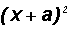or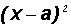equals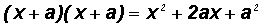equalsIMPORTANT: Notice that the third term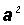of either of the two trinomials above is found by dividing the coefficient 2a of the second term (x-term) by 2 and raising the result to the second power

The Square Completion Strategy Given a Quadratic Equation in Two Variables

1. Isolateoron one side of the equation.
2. If necessary, change the coefficient of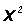to 1.
3. Findby dividing the coefficient of the x-term, which is 2a, by 2 and raising the result to the second power.
4. You then addto both sides of the equation!  This ensures that equality is preserved.  See WARNING below!

WARNING:  Should you have changed the coefficient ofby factoring out a number, say k, you must add/subtract!

Example 1:

Given the equationcomplete the square on the right side

Step 1:

Isolate the terms containing x on one side of the equation.

y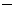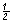=x2x

Step 2:

Let's multiply both sides of the equation by2 to change the coefficient ofto 1.2y + 1 = x2 + 2x

Step 3:

Findby dividing the coefficient of the x-term, which is 2, by 2 and raising the result to the second power.

That is,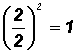Step 4:

We then add 1 form Step 3 to both sides of the equation!  This ensures that equality is preserved.2y + 1 + 1 = x2 + 2x + 12y + 2 = x2 + 2x + 1

and2y + 2 = (x + 1)2

As requested in the instructions, we completed the square on the right side of the equation.

Example 2:

Given the equation x = 2y212y + 7 complete the square on the right side.

Please note that we will use the above strategy, however, we will separate the y-terms from the x and the constant.

Step 1:

Isolate the terms containing y on one side of the equation.

x7 = 2y212y

Step 2:

Let's factor 2 out of the right side to change the coefficient of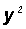to 1.

x7 = 2(y26y)

Step 3:

Findby dividing the coefficient of the y-term, which is6, by 2 and raising the result to the second power.

That is,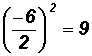.

Step 4:

So do we now add 9 from Step 3 to both sides of the equation? NO!

Please note that we CANNOT just add a 9 to the left side. This would change the value of the expression!  Actually we must add 2(9) because that's what we did on the right side too! Please study the following equation carefully!

x7 + 2(9) = 2(y26y + 9)

Are you noticing that we actually added 2(9) to the right side?

and x + 11 = 2(y3)2

As requested in the instructions, we completed the square on the right side of the equation.